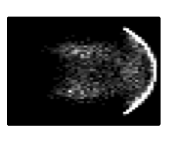# Universal Rephasing Dynamics after a Quantum Quench via Sudden Coupling of Two Initially Independent CondensatesWe consider a quantum quench in which two initially independent condensates are suddenly coupled and study the subsequent “rephasing” dynamics. For weak tunneling couplings, the time evolution of physical observables is predicted to follow universal scaling laws, connecting the short-time dynamics to the long-time nonperturbative regime. We first present a two-mode model valid in two and three dimensions and then move to one dimension, where the problem is described by a gapped sine-Gordon theory. Combining analytical and numerical methods, we compute universal time-dependent expectation values, allowing a quantitative comparison with future experiments.

1. Dalla Torre, E. G., Demler, E. & Polkovnikov, A. Universal Rephasing Dynamics after a Quantum Quench via Sudden Coupling of Two Initially Independent Condensates. Phys. Rev. Lett. 110, 090404 (2013).

10.1103/PhysRevLett.110.090404